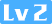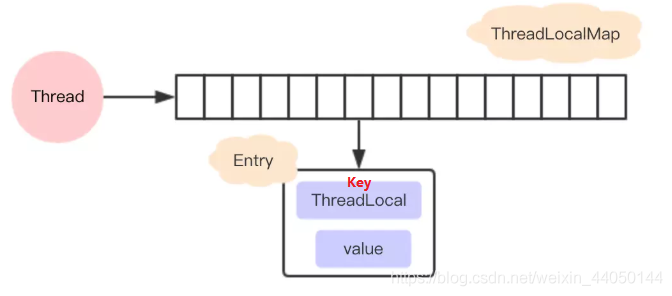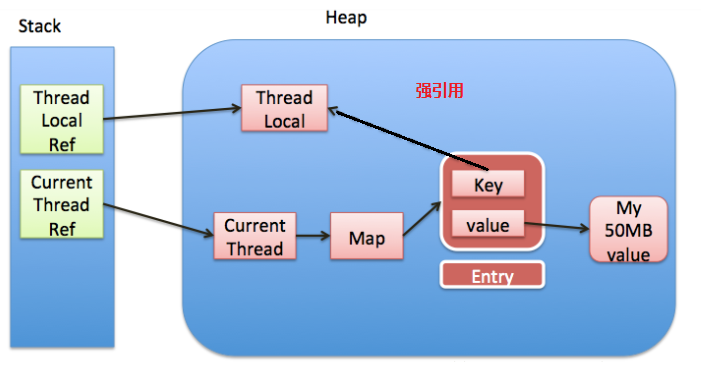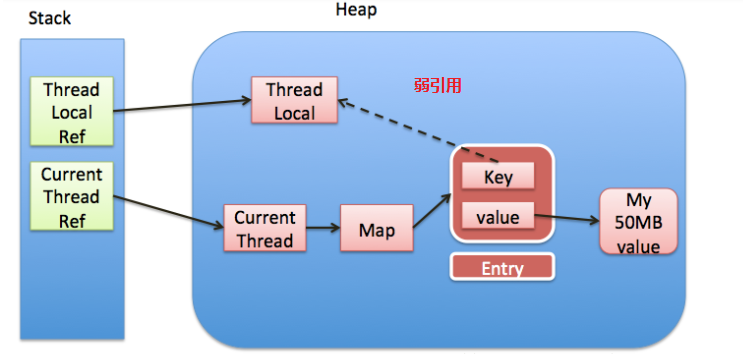待兔1491 0 0set方法：

``````  public void set(T value) {
//获得当前线程
ThreadLocalMap map \= getMap(t);//得到实际存储的map if (map != null)如果map已经存在，那么就存入
}``````

get方法：

``````/\*\*

\*/
public T get() {
ThreadLocalMap map \= getMap(t);//获得当前map if (map != null) {如果map存在
@SuppressWarnings("unchecked")
T result \= (T)e.value;//获得e中对应的value值。并返回 return result;
}
}
//会有两种情况执行当前代码
1.map不存在，
}``````
``````private T setInitialValue() {
T value \= initialValue();//调用initialValue获取初始化的值，此方法可以被子类重写，如果不重写默认返回null
ThreadLocalMap map \= getMap(t);//获得map if (map != null)如果map存在，那么直接set，则对应上面的第二种情况
map.set(this, value); else//对应上面的第一种情况 createMap(t, value);//那么对map初始化创建，将t（当前线程）和value作为第一个entry存放到map中。 return value;
}``````

remove方法：

``````删除当前线程中保存的ThreadLocal对应的实体entry
public void remove() {
m.remove(this);
}``````
``````private void remove(ThreadLocal<?> key) {
Entry\[\] tab \= table; int len = tab.length; int i = key.threadLocalHashCode & (len-1);//计算索引 for (Entry e = tab\[i\];
e != null;
e \= tab\[i = nextIndex(i, len)\]) {//进行线性探索，查找正确的key if (e.get() == key) {
e.clear();//调用弱引用的claer（）清除引用，
expungeStaleEntry(i);//然后连续段清除。 return;
}
}
}``````

``````void createMap(Thread t, T firstValue) {
}``````
``````ThreadLocalMap(ThreadLocal<?> firstKey, Object firstValue) {
table \= new Entry\[INITIAL\_CAPACITY\]; int i = firstKey.threadLocalHashCode & (INITIAL\_CAPACITY - 1);
table\[i\] \= new Entry(firstKey, firstValue);
size \= 1;
setThreshold(INITIAL\_CAPACITY);
}``````

``````  private static int nextIndex(int i, int len) { return ((i + 1 < len) ? i + 1 : 0);
} /\*\* \* Decrement i modulo len. \*/
private static int prevIndex(int i, int len) { return ((i - 1 >= 0) ? i - 1 : len - 1);
}``````
`````` private void set(ThreadLocal<?> key, Object value) { // We don't use a fast path as with get() because it is at // least as common to use set() to create new entries as // it is to replace existing ones, in which case, a fast // path would fail more often than not.
Entry\[\] tab \= table; int len = tab.length; int i = key.threadLocalHashCode & (len-1);//计算索引位置 for (Entry e = tab\[i\];
e != null;
e \= tab\[i = nextIndex(i, len)\]) {//根据获取到的索引进行循环，如果当前索引上的tab\[i\]不为空，在没有retuen的情况下，就使用nextIndex()获取下一个。也就是线性探测法
ThreadLocal<?> k = e.get();//这也就是tab\[i\]的key if (k == key) {判断是否与方法参数key相同，如果相同就替换value，然后return
e.value \= value; return;
} if (k == null) {//key为null，但是值不为null，说明之前的ThreadLocal对象已经被回收了，那么当前数组中的Entry是一个陈旧的元素
replaceStaleEntry(key, value, i);//用新元素替换陈旧的元素，这个方法进行了不少的垃圾清理动作，防止内存泄露。 return;
}
}

tab\[i\] \= new Entry(key, value);//ThreadLocal对应的key不存在并且没有找到陈旧的元素，则在空元素的位置创建一个新的Entry。 int sz = ++size;
//　cleanSomeSlots用于清除那些e.get()==null的元素，
// 这种数据key关联的对象已经被回收，所以这个Entry(table\[index\])可以被置null。
// 如果没有清除任何entry,并且当前使用量达到了负载因子所定义(长度的2/3)，那么进行 if (!cleanSomeSlots(i, sz) && sz >= threshold)
rehash();
}``````

1.1在循环里会进行判断，tab[i].get,就是table[i]的key，是否与方法参数key相同，相同就替换value，然后return

1.2如果不相同再判断entry的key是否为null，如果是null的话说明这个位置被回收了，那么调用replaceStaleEntry(key,value,i)方法，也就是替换无效的entry（那么再这个无效的table[i]处可以用新的key-value进行替换，并清楚其他无效的entry）。然后return。

2.如果循环结束了，说明当前table[i]为null，那就直接在这个位置放entry就ok了，然后size++；

3.最后进行判断，如果没有清楚任何一个entry并且当前size已经大于扩容因子了，也就是数组的2/3，那就需要rehash。

`````` private void replaceStaleEntry(ThreadLocal<?> key, Object value, int staleSlot) {
Entry\[\] tab \= table;//entry数组 int len = tab.length;
Entry e;//entry // Back up to check for prior stale entry in current run. // We clean out whole runs at a time to avoid continual // incremental rehashing due to garbage collector freeing // up refs in bunches (i.e., whenever the collector runs).
int slotToExpunge = staleSlot;//之后用于清理的起点 for (int i = prevIndex(staleSlot, len);//这里是向staleSlot前扫描，时刻记住此时的staleSlot是一个无效的entry。
(e \= tab\[i\]) != null;
i \= prevIndex(i, len)) if (e.get() == null)//向前扫描找到了第一个无效的entry。那么起点就是这个无效的entry，否则起点就是最开始的staleSlot
slotToExpunge \= i; // Find either the key or trailing null slot of run, whichever // occurs first
for (int i = nextIndex(staleSlot, len);//接着向后扫描
(e \= tab\[i\]) != null;
i \= nextIndex(i, len)) {
ThreadLocal<?> k = e.get(); // If we find key, then we need to swap it // with the stale entry to maintain hash table order. // The newly stale slot, or any other stale slot // encountered above it, can then be sent to expungeStaleEntry // to remove or rehash all of the other entries in run.
if (k == key) {//如果相等，那么更新value即可
e.value \= value;这时候e就是一个有效的entry，

tab\[i\] \= tab\[staleSlot\];//然后这时候把无效的赋值到当前i位置
tab\[staleSlot\] \= e;//再把这个entry赋值给最开始传入这个方法的位置处。也就是交换了位置。让无效的entry尽可能靠后。 // Start expunge at preceding stale entry if it exists
if (slotToExpunge == staleSlot)//如果向前找没有找到无效的entry，那么开始的起点就是i。也就是交换后的无效的位置。
slotToExpunge \= i;
cleanSomeSlots(expungeStaleEntry(slotToExpunge), len); return;
} // If we didn't find stale entry on backward scan, the // first stale entry seen while scanning for key is the // first still present in the run.
if (k == null && slotToExpunge == staleSlot)//这里就是如果向前查找没有无效的entry，然后当前向后扫描的entry无效，则更新清理起点。
slotToExpunge \= i;
} // If key not found, put new entry in stale slot
tab\[staleSlot\].value = null;//上面的k==key判断没有经历到的话，那么说明没有找到key，有也就是说key之前不存在，那么直接再最开始的无效entry，也就是tab\[stableSlot\]上新增即可
tab\[staleSlot\] \= new Entry(key, value); // If there are any other stale entries in run, expunge them
if (slotToExpunge != staleSlot)//经过上面的for循环之后到这，说明存在其他的无效entry需要进行清理。
cleanSomeSlots(expungeStaleEntry(slotToExpunge), len);
}``````

``````private int expungeStaleEntry(int staleSlot) {//连续段清除
Entry\[\] tab \= table; int len = tab.length; // expunge entry at staleSlot
tab\[staleSlot\].value = null;//清理无效entry，置空
tab\[staleSlot\] \= null;
size\--;//size减1，置空后table的被使用量减1 // Rehash until we encounter null
Entry e; int i; for (i = nextIndex(staleSlot, len);
(e \= tab\[i\]) != null;
i \= nextIndex(i, len)) {//从staleSlot开始向后扫描一段连续的entry
ThreadLocal<?> k = e.get(); if (k == null) {//如果遇到key为null，表示无效entry，进行清理
e.value \= null;
tab\[i\] \= null;
size\--;
} else {//如果key不为null，计算索引 int h = k.threadLocalHashCode & (len - 1); if (h != i) {计算出来的索引h与当前所在位置的索引i不一致，那么就置空当前的tab\[i\]，
tab\[i\] \= null; // Unlike Knuth 6.4 Algorithm R, we must scan until // null because multiple entries could have been stale.
while (tab\[h\] != null)//然后从h开始向后线性探测到第一个空的slot，把e赋值过去。
h \= nextIndex(h, len);
tab\[h\] \= e;
}
}
} return i;//下一个为空的slot索引。
}``````

``````//启发式的扫描清楚，扫描次数由传入的参数n决定。
//从i开始向后扫描，（不包括i，因为上面已经说了，i所对应的entry是null）
//n控制扫描次数，正常情况下为log2（n），如果找到了无效entry，会将n重置为table的长度len，然后再调用上面的方法进行连续段清除。
private boolean cleanSomeSlots(int i, int n) { boolean removed = false;
Entry\[\] tab \= table; int len = tab.length; do {
i \= nextIndex(i, len);
Entry e \= tab\[i\]; if (e != null && e.get() == null) {
n \= len;//这里就是找到了一个无效的entry，那么重置n，并段清除。
removed \= true;
i \= expungeStaleEntry(i);
}
} while ( (n >>>= 1) != 0);//无符号的右移动，可以用于控制扫描次数在log2（n） return removed;
}``````

`````` private void rehash() {
expungeStaleEntries();//全清理 // Use lower threshold for doubling to avoid hysteresis
//threshold = 2/3\*len,所以-threshold / 4=len/2.这里主要是因为上面做了一次全清理所以减少，需要进行判断。判断的时候把阈值减少了。
if (size >= threshold - threshold / 4)
resize();
}``````
`````` private void expungeStaleEntries() {
Entry\[\] tab \= table; int len = tab.length; for (int j = 0; j < len; j++) {
Entry e \= tab\[j\]; if (e != null && e.get() == null)
expungeStaleEntry(j);
}
}``````
`````` private void resize() {
Entry\[\] oldTab \= table; int oldLen = oldTab.length; int newLen = oldLen \* 2;//扩容，扩为原来的两倍，这样保证了长度为2的幂
Entry\[\] newTab \= new Entry\[newLen\]; int count = 0; for (int j = 0; j < oldLen; ++j) {
Entry e \= oldTab\[j\]; if (e != null) {
ThreadLocal<?> k = e.get(); if (k == null) {
e.value \= null; // Help the GC//虽然做过一次清理，但在扩容的时候可能会又存在key==null的情况
} else { int h = k.threadLocalHashCode & (newLen - 1);//同样用线性探测法来设置每个位置。 while (newTab\[h\] != null)
h \= nextIndex(h, newLen);
newTab\[h\] \= e;
count++;
}
}
}

setThreshold(newLen);//设置新的阈值
size \= count;
table \= newTab;
}``````

``````private Entry getEntry(ThreadLocal<?> key) { int i = key.threadLocalHashCode & (table.length - 1);//根据key计算索引，获取entry
Entry e \= table\[i\]; if (e != null && e.get() == key)//如果这个table\[i\]不为null且其key等于key，就返回entry return e; else
return getEntryAfterMiss(key, i, e);//如果不是，那就执行这个函数
}``````
``````private Entry getEntryAfterMiss(ThreadLocal<?> key, int i, Entry e) {
Entry\[\] tab \= table; int len = tab.length; while (e != null) {
ThreadLocal<?> k = e.get(); if (k == key) return e; if (k == null)
expungeStaleEntry(i);//清除无效的entry else i \= nextIndex(i, len);//基于线性探测法向后扫描
e \= tab\[i\];
} return null;//如果都没有就返回null
}``````1.没有手动删除这个Entry